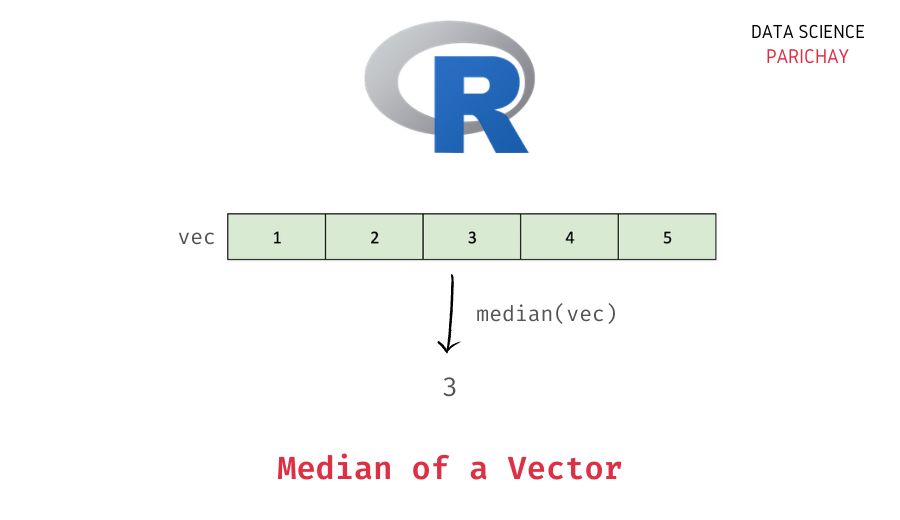# Calculate Median of a Vector in R

A vector is a one-dimensional data structure used to store data of the same type in R. Numeric vectors are commonly used to store a sequence of numbers. In this tutorial, we will look at how to get the median value of a numeric vector in R with the help of some examples.

## What is the median of a distribution?

Median is a descriptive statistic used as a measure of central tendency in a distribution. It gives a better idea of the center of the distribution (than other estimates such as the mean) particularly if the data has outliers.

The median of a group of values is the middle value, that is, the value that divides the distribution into two equal halves. To manually compute the median, you can sort the values and choose the middle value as the median.

## How to get the median of a vector in R?

You can use the R `median()` function to get the median of values in a vector. Pass the vector as an argument to the function. The following is the syntax –

```# median of values in a vector
median(x, na.rm=FALSE)```

The following are the arguments that you can give to the `median()` function in R.

• x – The vector for which you want to compute the median.
• na.rm – (Optional argument) Indicates whether to remove the missing values before computing the median. It is `FALSE` by default.

The function returns the median of the passed vector.

## Examples

Let’s look at some examples of using the above method to compute the median of values in a vector.

### Median of a numeric vector

Let’s create a vector of numbers (and without any `NA` values) and apply the `median()` function.

📚 Data Science Programs By Skill Level

Introductory

Intermediate ⭐⭐⭐

🔎 Find Data Science Programs 👨‍💻 111,889 already enrolled

Disclaimer: Data Science Parichay is reader supported. When you purchase a course through a link on this site, we may earn a small commission at no additional cost to you. Earned commissions help support this website and its team of writers.

```# create a vector
vec <- c(1, 3, 2, 4, 5)
# median of values in the vector
median(vec)```

Output:

`3`

We get the median as 3, which is the middle value in the above vector of numbers

What would happen if there are some `NA` present values in the vector?

Let’s find out.

First, we will create a vector with some `NA` values and then apply the `median()` function without any additional arguments.

```# create a vector with NA values
vec <- c(1, 3, NA, 2, 4, 5, NA)
# median of values in the vector
median(vec)```

Output:

`<NA>`

You can see that we get `NA` as the output. This is because comparing anything with `NA` results in an `NA` in R.

### Median of a vector with `NA` values

You can pass `TRUE` to the `na.rm` parameter of the `median()` function to exclude missing values when computing the median of a vector.

```# create a vector with NA values
vec <- c(1, 3, NA, 2, 4, 5, NA)
# median of values in the vector
median(vec, na.rm = TRUE)```

Output:

`3`

Now we get the median of the values in the above vector as 3.

You might also be interested in –

•